## NCERT Solutions for Class 12 Physics Chapter 11 Dual Nature of Radiation and Matter

NCERT Solutions for Class 12 Physics Chapter 11 Dual Nature of Radiation and Matter are part of NCERT Solutions for Class 12 Physics. Here we have given. NCERT Solutions for Class 12 Physics Chapter 11 Dual Nature of Radiation and Matter

 Board CBSE Textbook NCERT Class Class 12 Subject Physics Chapter Chapter 11 Chapter Name Dual Nature of Radiation and Matter Number of Questions Solved 37 Category NCERT Solutions

Question 1.
Find the
(a) maximum frequency, and
(b) minimum wavelength of X-rays produced by 30 kV electrons.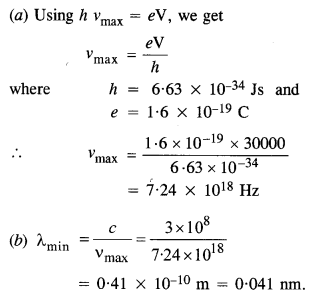Question 2.
The work function of caesium metal is 2.14 eV. When light of frequency 6 x 1014 Hz is incident on the metal surface, photo emission of electrons occurs. What is the
(а)   maximum kinetic energy of the emitted electrons,
(b)   stopping potential, and
(c) maximum speed of the emitted photo electrons ?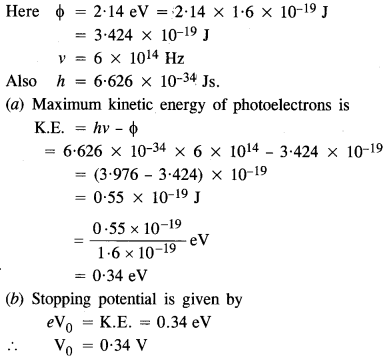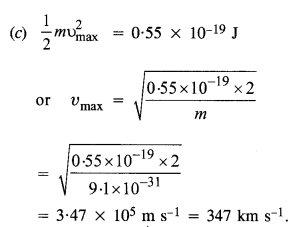Question 3.
The photoelectric cut-off voltage in a certain experiment is 1.5 V. What is the maximum kinetic energy of photoelectrons emitted?
V0 = 1.5 V
∴ Maximum kinetic energy of the emitted electrons,
$$\frac { 1 }{ 2 } m{ V }_{ max }^{ 2 }=e{ V }_{ 0 }=1.5j=15eV$$

Question 4.
Monochromatic light of wavelength 632.8 nm is produced by a helium-neon laser. The power emitted is 9.42 mW.
(a) Find the energy and momentum of each photon in the light beam.
(b) How many photons per second, on average, arrive at a target irradiated by this beam? (Assume the beam to have a uniform cross-section which is less than the target area), and
(c) How fast does a hydrogen atom have to travel in order to have the same momentum as that of the photon?
Given, λ = 632.8 nm = 632.8 x 10-9m
Power, P = 9.42 mW = 9.42 x 10-3 W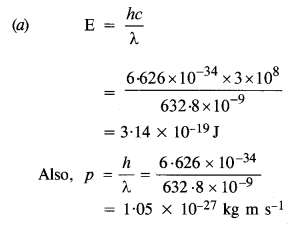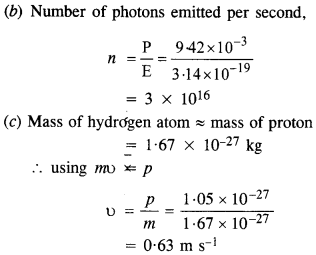Question 5.
The energy flux of sunlight reaching the surface of the earth is 1.388 x 103 W/m2. How many photons (nearly) per square meter are incident on the Earth per second? Assume that the photons in the sunlight have an average wavelength of 550 nm.Question 6.
In an experiment on the photoelectric effect, the slope of the cut-off voltage versus frequency of incident light is found to be 4.12 x 10-15 V s. Calculate the value of Planck’s constant.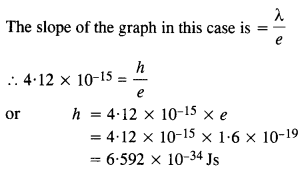Question 7.
A 100 W sodium lamp radiates energy uniformly in all directions. The lamp is located at the center of a large sphere that absorbs all the sodium light which is incident on it. The wavelength of the sodium light is 589 nm.
(a) What is the energy per photon associated with sodium light?
(b) At what rate are the photons delivered to the sphere?
Power of sodium lamp,
P = 100 W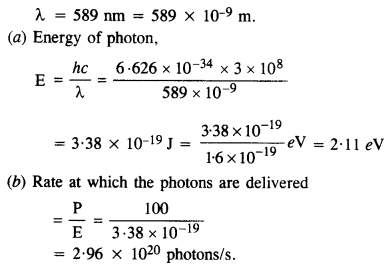Question 8.
The threshold frequency for a certain metal is 3.3 x 1014 Hz. If the light of frequency 8.2 x 1014 Hz is incident on the metal, predict the cut-off voltage for the photoelectric emission.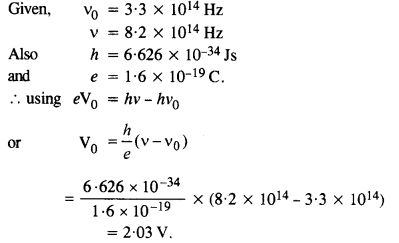Question 9.

The work function for a certain metal is 4.2 eV. Will this metal give photoelectric emission for incident radiation of wavelength 330 nm?Since the energy of incident radiation less than the work function (4.2 eV) of the metal, therefore, the photoelectric emission can not take place from the given metal. 11.10.

Question 10.
Light of frequency 7.21 x 1014 Hz is incident on a metal surface. Electrons with a maximum speed of 6.0 x 105 m/s are ejected from the surface. What is the threshold frequency for the photoemission of electrons?
Using the relation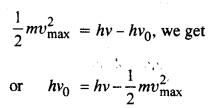Question 11.
Light of wavelength 488 nm is produced by an argon laser which is used in the photoelectric effect. When light from this spectral line is incident on the cathode, the stopping (cut-off) potential of photoelectrons is 0.38 V. Find the work function of the material from which the cathode is made.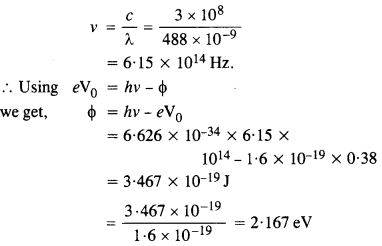Question 12.
Calculate the
(a) momentum, and
(b) de Broglie wavelength of the electrons accelerated through a potential difference of 56 V.
Given V = 56 V.
(a) the momentum of the electron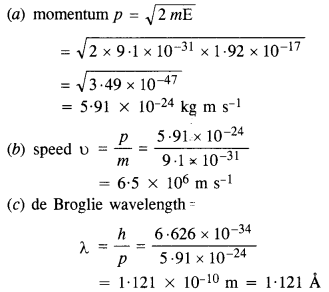Question 13.
What is the
(a) momentum,
(b) speed, and
(c) de Broglie wavelength of an electron with the kinetic energy of 120 eV?
Here, E = 120 eV = 120 x 1.6 x 10-19J
= 1.92 x 10-17 JQuestion 14.
The wavelength of light from the spectral emission line of sodium is 589 nm. Find the kinetic energy at which
(a) an electron, and
(b) a neutron would have the same de Broglie wavelength.Question 15.
What is the de Broglie wavelength of
(a) a bullet of mass 0.040 kg travelling at the speed of 1.0 km/s,
(b) a ball of mass 0.060 kg moving at a speed of 1.0 m/s, and
(c) a dust particle of mass 1.0 x 10-9 kg drifting with a speed of 2.2 m/s?
(a) Here, m = 0.040 kg and υ = 1.0 km/s .
= 1000 m/s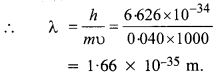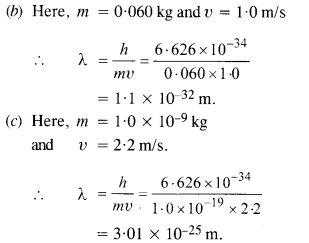Question 16.
An electron and a photon each have a wavelength of 100 nm. Find
(a) their momenta,
(b) the energy of the photon, and
(c) the kinetic energy of electrons.Question 17.

(a) For what kinetic energy of a neutron will the associated de Broglie wavelength be
1.40 x 10-10 m?  (C.B.S.E. 2008)
(b) Also find the de Broglie wavelength of a neutron, in thermal equilibrium with matter, having an average kinetic energy of $$\frac { 3 }{ 2 }$$ kT at 300 K.
(a) Here λ = 1.40 x 10-10m
Also, h = 6.63 X 10-34 Js
and m = 1.67 x 10-27kg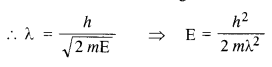Question 18.

Show that the wavelength of electromagnetic radiation is equal to the de Broglie wavelength of its quantum (photon).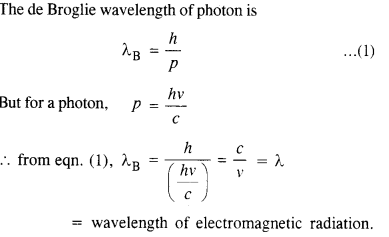Question 19.

What is the de Broglie wavelength of a nitrogen molecule in air at 300 K? Assume that the molecule is moving with the root mean square speed of molecules at this temperature. (Atomic mass of nitrogen = 14.0076 u)
For nitrogen,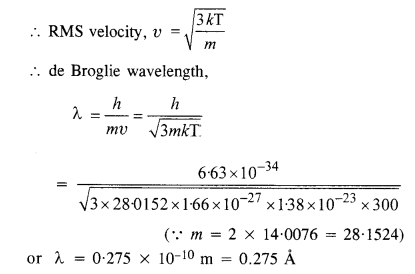Question 20.

(a) Estimate the speed with which electrons emitted from a heated cathode of an evacuated tube impinge on the anode maintained at a potential difference of 500 V with respect to the cathode. Ignore the small initial speeds of the electrons. The ‘specific charge’ of the electron i.e., its elm is given to be 1.76 x 1011 C kg-1.
(b) Use the same formula you employ in
(a) to obtain electron speed for an anode potential of 10 MV. Do you see what is wrong? In what way is the formula to be modified?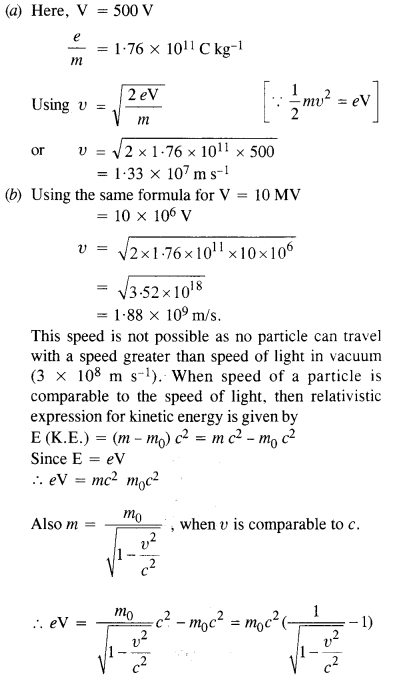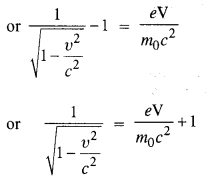Question 21.

(a) A monoenergetic electron beam with an electron speed of 5.20 x 106 ms-1 is subject to a magnetic field of l.30 x 10-4 T normal to the beam velocity. What is the radius of the circle traced by the beam, given elm for electron equals 1.76 X 1011 C kg-1?
(b) Is the formula you employ in
(a) valid for calculating radius of the path of a 20 MeV electron beam? If not, in what way is it modified?
(a) υ  = 5.20 x 106ms_1
B = 1.30 x 10 4T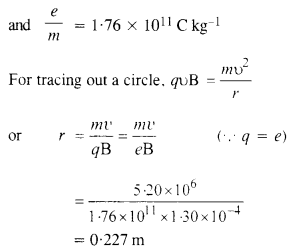(b) The formula employed in part (a) is not valid because with the increase in velocity, mass varies and in the above formula we have taken m as constant. Instead, mQuestion 22.
An electron gun with its anode at a potential of 100 V fires out electrons in a spherical bulb containing hydrogen gas at low pressure (~ 10-2 mm of Hg). A magnetic field of 2.83 x 10 4 T curves the path of the electrons in a circular orbit of radius 12.0 cm. (The path can be viewed because the gas ions in the path focus the beam by attracting electrons, and emitting light by electron capture; this method is known as the ‘fine beam tube’ method.) Determine e/m from the data.Question 23.
(a) An X-ray tube produces a continuous spectrum of radiation with its short wavelength end at (45 Å. What is the maximum energy of a photon in the radiation?
(b) From your answer to (a), guess what order of accelerating voltage (for electrons) is required in such a tube.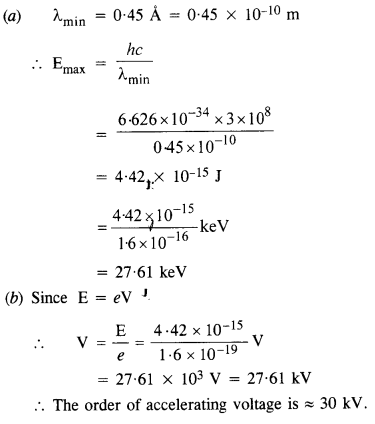Question 24.
In an accelerator experiment on high-energy collisions of electrons with positrons, a certain event is interpreted as the annihilation of an electron-positron pair of total energy 10.2 BeV into two y-rays of equal energy. What is the wavelength associated with each γ-ray?   (1 BeV = 109 eV)
The energy carried by the pair of γ-rays = 10 .2 BeV
The energy of each γ ray is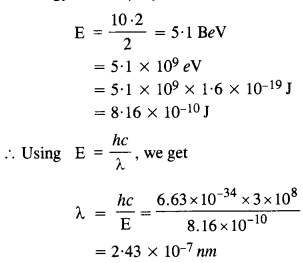Question 25.
Estimating the following two numbers should be interesting. The first number will tell you why radio engineers do not need to worry much about photons! The second number tells you why our eye can never ‘count photons’, even in barely detectable light.
(a) The number of photons emitted per second by a Mediumwave transmitter of 10 kW power, emitting radio waves of wavelength 500 m.
(b) The number of photons entering the pupil of our eye per second corresponding to the minimum intensity of white light that we humans can perceive (~ 10_1° W m-2). Take the area of the pupil to be about 0.4 cm2, and the average frequency of white light to be about 6 x 1014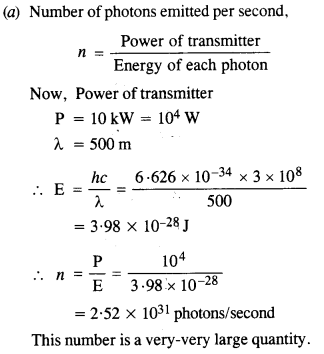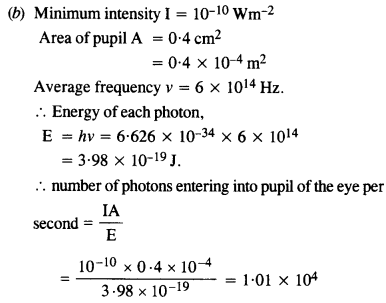This is quite a small number, but still large enough to be counted.
Comparison of cases (a) and (b) tells us that our eyes can not count the number of photons individually.

Question 26.
Ultraviolet light of wavelength 2271 A from a 100 W mercury source irradiates a photo-cell made of molybdenum metal. If the stopping potential is -1.3 V, estimate the work function of the metal. How would the photo-cell respond to a high intensity
(~ 10s W m-2) red light of wavelength 6328 A produced by a He-Ne laser?
[C.B.S.E. 2005, UC, 13]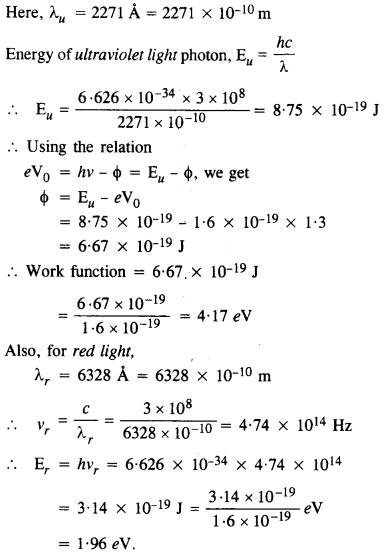Since the energy of a red photon is less than the work function for the metal, the photocell does not respond to red light.

Question 27.
Monochromatic radiation of wavelength 640.2 nm (1 nm = 10-9 m) from a neon lamp irradiates photosensitive material made of caesium or tungsten. The stopping voltage is measured to be 0.54 V. The source is replaced by an iron source and its 427.2 nm line irradiates the same photo-cell. Predict the new stopping voltage.Question 28.
A mercury lamp is a convenient source for studying the frequency dependence of photoelectric emission since it gives a number of spectral lines ranging from the UV to the red end of the visible spectrum. In our experiment with rubidium photo-cell, the following lines from a mercury source were used :
λx = 3650 Å, λ2 = 4047 Å, λ3 = 4358 Å, λ4 = 5461 Å, λ5 = 6907 Å.
The stopping voltages, respectively, were measured to be :
V01 = 1.28 V, V02 = 0.95 V, f03 = 0.74 V, = 0.16 V,V05 = 0 V
(a) Determine the value of Planck’s constant
(b) Estimate the threshold frequency and work function for the material.
(a) From the Einstein photoelectric equation,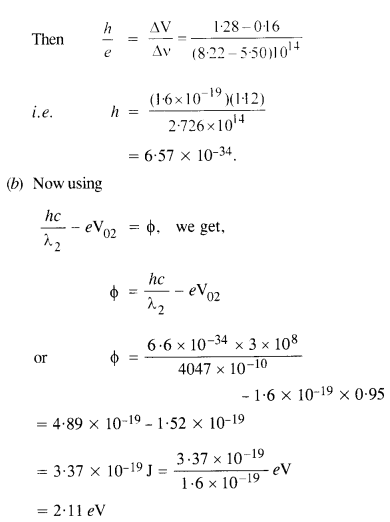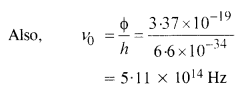Question 29.

The work function for the following metals is given : Na : 2.75 eV ; K : 2.30 eV ; Mo : 417 eV ; Ni: 515 eV. Which of these metals will not give photoelectric emission for a radiation of wavelength 3300 Å from a He-Cd laser placed 1 m away from the photocell? What happens if the laser is brought nearer and placed 50 cm away? (C.B.S.E. 2009)
Here, λ = 3300 A = 3300 x 10-10 m
Distance, r’ = 1 m and r’ = 50 cm = 0.5 m
Using the relationSince the energy E of the incident photon of light is less than the work functions of Mo and Ni metals, so photoelectric emission will not occur in Mo and Ni. The distance of the source does not increase or decrease the energy of the photon of the light incident, therefore, the energy of electrons ejected will not change but the intensity of ejected electrons will increase (1 α 1/r2 ) and become four times.

Question 30.
Light of intensity 10-5 W m-2 falls on a sodium photo­cell of surface area 2 cm2. Assuming that the top 5 layers of sodium absorb the incident energy, estimate time required for photoelectric emission in the wave- picture of radiation. The work function for the metal is given to be about 2 eV. What is the implication of your answer?
A = 2 cm2 = 2 x 10-4 m2
φ = 2eV = 2 x 1.6 x 10-19 J
= 3.2 X 10-19  J.
Taking the approximate radius of an atom as 10-10 m. the effective area of sodium atom is ≈ r2 = 10-20 m.
.’. If there is one free electron per atom, then the number of electrons in five layers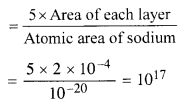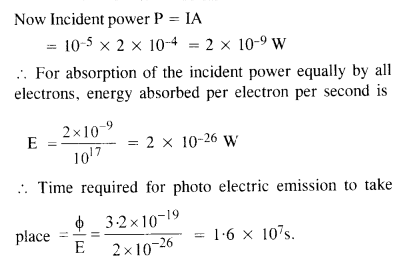The answer obtained implies that the time of emission of the electron is very large and is not in agreement with the observed time of emission, which is approximately 10 9 s. Thus wave-picture of radiation is not applicable for photo-electric emission.

Question 31.
Crystal diffraction experiments can be performed using X-rays, or electrons accelerated through appropriate voltage. Which probe has greater energy? (For quantitative comparison, take the wavelength of the probe equal to 1 Å which is of the order of interatomic spacing in the lattice) (me = 9.11 x 10­-31 kg).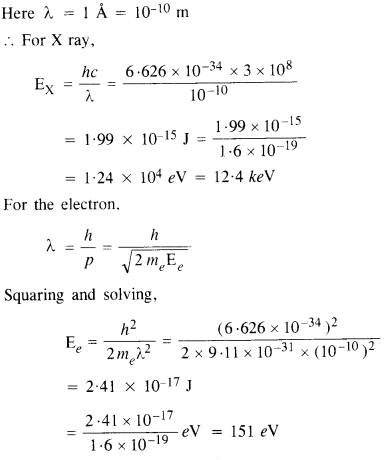Clearly, the energy of photons is much greater than the energy of electrons.

Question 32.
(a) Obtain the de Broglie wavelength of a neutron of kinetic energy 150 eV. As you have seen in Exercise 44, an electron beam of this energy is suitable for crystal diffraction experiments. Would a neutron beam of the same energy be equally suitable?
Explain. (mn = 1.675 x 10-27 kg).
(b) Obtain the de Broglie wavelength associated with thermal neutrons at room temperature (27°C). Hence explain why a fast neutron beam needs to be thermalized with the environment before it can be used for neutron diffraction experiments.This wavelength is about hundred times smaller than the interatomic separation of crystals. Thus, neutrons are not suitable for diffraction experiments in case of crystals.This wavelength is comparable to the interatomic spacing of crystals. Therefore, thermal electrons are able to interact with the crystal. Sinceincreasing the temperature, decreases their de Broglie wavelength and they become unsuitable for crystal diffraction. Thus, the fast beam of neutrons needs to be thermalised with the environment for neutron diffraction experiment.

Question 33.
An electron microscope uses electrons accelerated by a voltage of 50 kV. Determine the de Broglie wavelength associated with the electrons. If other factors (such as numerical aperture, etc.) are taken to be roughly the same, how does the resolving power of an electron microscope compare with that of an optical microscope which uses yellow
light ?Question 34.

The wavelength of a probe is roughly a measure of the size of a structure that it can probe in some detail. The quark structure of protons and neutrons appears at the minute length-scale of 10-15 m or less. This structure was first probed in early 1970’s using high-energy electron beams produced by a linear accelerator at Stanford, USA. Guess what might have been the order of energy of these electron beams. (Rest mass energy of electron = 0.511 MeV).Thus, the energy of the proton ejected out of the linear accelerator is of the order of BeV.

Question 35.
Find the typical de Broglie wavelength associated with a He atom in helium gas at room temperature (27 °C) and 1 atm pressure, and compare it with the mean separation between two atoms under these conditions.
Here T = 27 °C = 27 + 273 = 300 K
P = 1 atm = 1.01 x 105 Nm-2
Also, the mass of helium atom,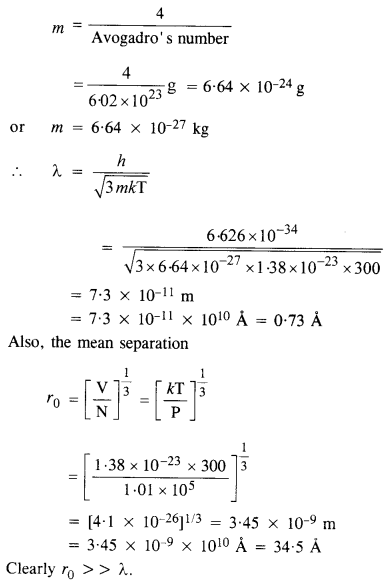Question 36.

Compute the typical de Broglie wavelength of an electron in metal at 27°C and compare it with the mean separation between two electrons in a metal which is given to be about 2 x 10_10 m.
Here, T = 27°C = 27 + 273 = 300 KQuestion 37.

(a) Quarks inside protons and neutrons are thought to carry fractional charges [(+2/3) e; (- l/3)e]. Why do they not show up in *Millikan’s oil-drop experiment?
(b) What is so special about the combination elm? Why do we not simply talk of e and m separately?
(c) Why should gases be insulators at ordinary pressures and start conducting at very low pressures?
(d) Every metal has a definite work function. Why do all photoelectrons not come out with the same energy if incident radiation is monochromatic? Why is there an energy distribution of photoelectrons?
(e) The energy and momentum of an electron are related to the frequency and wavelength of the associated matter wave by the relations :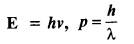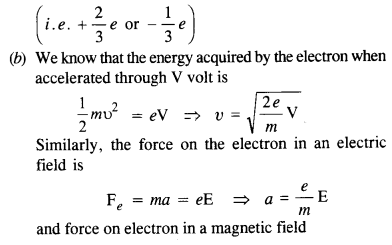Similarly energy E = hv = $$\frac { 1 }{ 2 }$$ m(λv)2 is also constant for a given matter wave so phase λv is also not physically significant.